# Apply the Pattern to Find the Missing Symbol

In this worksheet, students will find the missing symbol to complete a grid of nine boxes. It will develop their ability to find and follow patterns in shapes and symbols.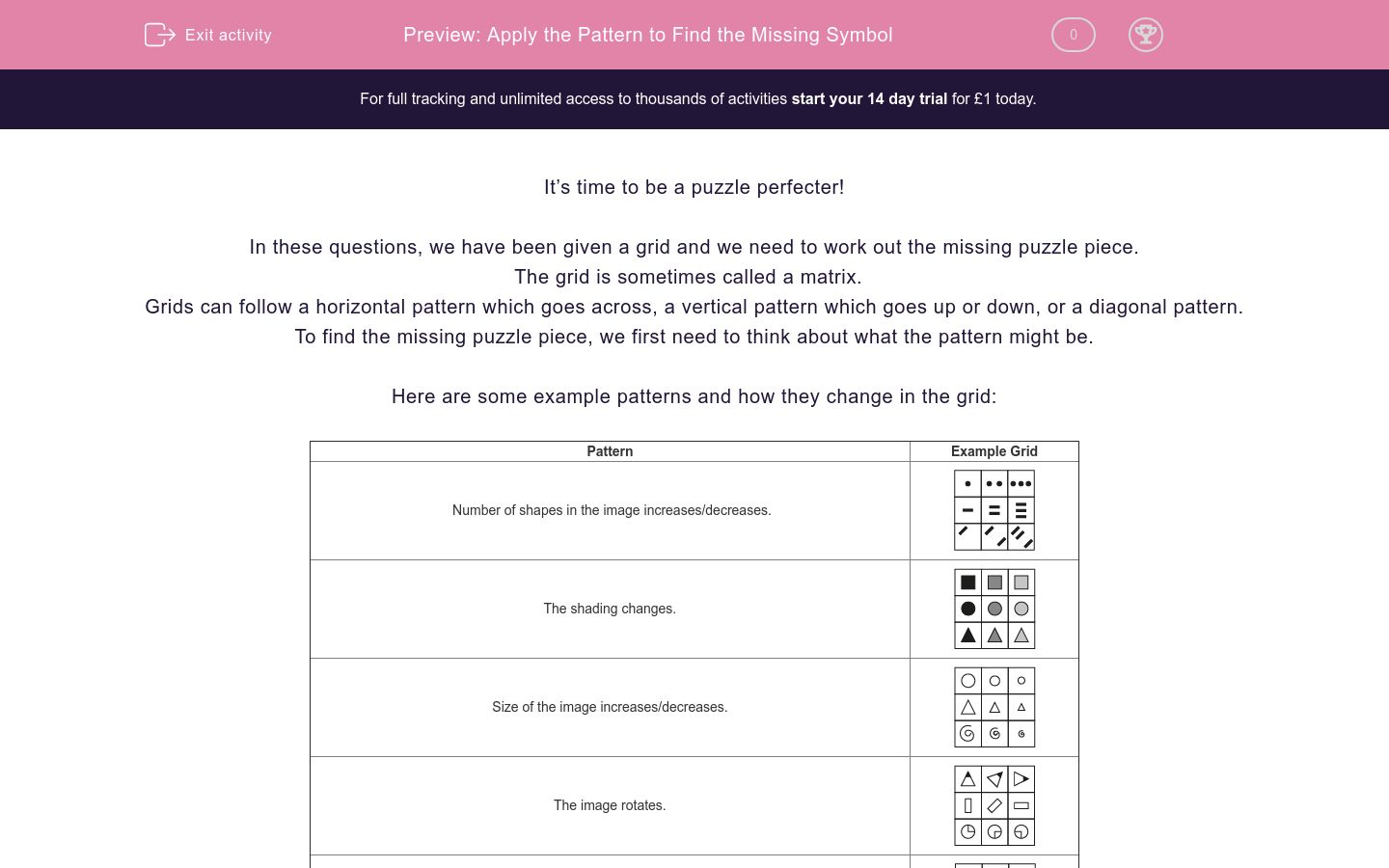Key stage:  KS 2

Curriculum topic:   Spatial and Non-Verbal Reasoning

Curriculum subtopic:   Complete the Grid

Difficulty level:### QUESTION 1 of 10

It’s time to be a puzzle perfecter!

In these questions, we have been given a grid and we need to work out the missing puzzle piece.

The grid is sometimes called a matrix.

Grids can follow a horizontal pattern which goes across, a vertical pattern which goes up or down, or a diagonal pattern.

To find the missing puzzle piece, we first need to think about what the pattern might be.

Here are some example patterns and how they change in the grid:

 Pattern Example Grid Number of shapes in the image increases/decreases.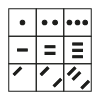The shading changes.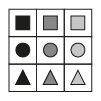Size of the image increases/decreases.The image rotates.Shapes within the symbol change position.The number of sides of the shapes in the image changes.Take a look at this example:Which of the following images would complete this grid?

a)b)c)d)The pattern in this case is horizontal.

The pattern is that the number of sides of each shape increases by one each time. Also, the centre shape gets slightly larger each time. Therefore the answer is b.

It’s now your turn to find the missing puzzle piece in grids. Remember to decide if it is horizontal, vertical or diagonal, when working out what the pattern is.

Good luck puzzle champ!

Always rule out any obviously incorrect answers.

Before you find the missing image in this grid, look carefully at all the shapes here to make some initial observations.

Complete the table below to indicate how many of each shape you would expect to see in the missing square.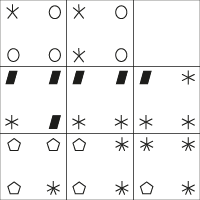0 1 2 3 Circle 5-Point Star Parallelogram 6-Point Star Pentagon 7-Point Star

Choose the image from the options below that best completes the grid.a)b)c)d)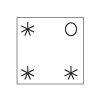e)Choose the image from the options below that best completes the grid.a)b)c)d)e)Choose the image from the options below that best completes the grid.a)b)c)d)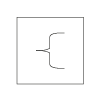e)Choose the image from the options below that best completes the grid.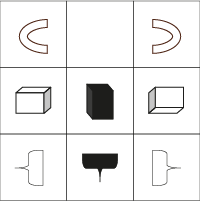a)b)c)d)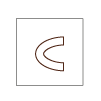e)Choose the image from the options below that best completes the grid.a)b)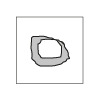c)d)e)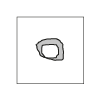Choose the image from the options below that best completes the grid.a)b)c)d)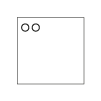e)Choose the image from the options below that best completes the grid.a)b)c)d)e)Choose the image from the options below that best completes the grid.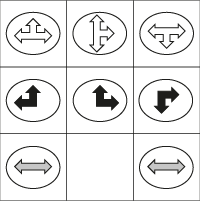a)b)c)d)e)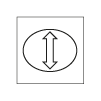Choose the image from the options below that best completes the grid.a)b)c)d)e)• Question 1

Before you find the missing image in this grid, look carefully at all the shapes here to make some initial observations.

Complete the table below to indicate how many of each shape you would expect to see in the missing square.0 1 2 3 Circle 5-Point Star Parallelogram 6-Point Star Pentagon 7-Point Star
EDDIE SAYS
Good try, puzzle perfecter! Using the shapes identified in this table hopefully helped you to start spotting the patterns in the grid. Did you observe that the missing image will have 1 circle and 3 5-pointed stars? This is because the number of stars increases by 1 each time and the number of circles decrease by 1 each time. There will be no parallelograms, 6-pointed stars, pentagons or 7-pointed stars in the correct option.
• Question 2

Choose the image from the options below that best completes the grid.e)EDDIE SAYS
In this question, you needed to be a total STAR detective! We were looking for a 5-pointed star to complete the grid pattern, which quickly leaves us with only options c and e to choose between. Now you need to look at the pattern in other parts of the grid to identify which to choose. In the second and third rows, the stars are increasing by 1 in an anti-clockwise direction. Therefore, the next star to be added in the missing grid square would be in the bottom right-hand corner of the four images. This means that option e is the correct answer, as it has a 5-pointed star in this location.
• Question 3

Choose the image from the options below that best completes the grid.d)EDDIE SAYS
How did you approach this one, puzzle perfecter? Did you identify that the missing image would need to be a black arrow, to match with the two squares above? This means that we can rule out options b and e immediately. The next step is to look at the directional pattern. In each column the arrows are moving in an anti-clockwise direction. The arrow in the bottom, right square will need to be pointing upwards. This makes option d the best fit.
• Question 4

Choose the image from the options below that best completes the grid.a)EDDIE SAYS
Good effort, puzzle perfecter! Option a is the best fit here. The shapes in each column remain the same, but they are rotating by a quarter turn (or 90 degrees) in a clockwise direction. So the missing square will show the same image as the middle, right square but rotated a quarter of a turn anti-clockwise. Did you spot the rotating pattern here? It's important to notice if the pattern is moving clockwise or anti-clockwise, and either follow or reverse this based on if the missing square is before or after the squares provided.
• Question 5

Choose the image from the options below that best completes the grid.e)EDDIE SAYS
Did you spot that the middle column only contains black images? This tells us that the missing image must be black, so we can forget about options a and d. The three images in each row are the same (horseshoe, rectangle, bracket) so we know that we are missing the horseshoe, which rules out option c. The shapes are rotating through a quarter turn (or 90 degrees) in the middle and bottom rows. Therefore, option e is the best fit as it shows a quarter turn clockwise from the shape on its left and a quarter turn anti-clockwise from the shape on its right. Wow, we really need to be confident with those clock directions in these rotation-focused questions!
• Question 6

Choose the image from the options below that best completes the grid.d)EDDIE SAYS
Each of the rows in this grid contains images of the same colour (black, grey or white), so we know that the missing image must be grey. This rules out option a and c immediately. If we focus on options b, d and e, we need to match the orientation of the grey images to those on the left and right of the missing square. The orientation means the shape's position, the direction in which it is pointing. The orientation of the images is not changing, which makes option d the best fit. Your detective's eyes may also have observed that the shapes in each row have three different sizes - small, medium and large. If we had been given two options of the same orientation but different sizes, then we would need to have chosen the smaller of the two. Great detective work so far - keep it up in the remaining questions!
• Question 7

Choose the image from the options below that best completes the grid.d)EDDIE SAYS
Some useful things to spot here are that column one has 3 objects in each box, column two has 1, and column three has 2 - so the missing image has 2 objects in it. This rules out options a and c. You may also spot that each row and column has one box with grey lines, another with black arrows, and one with white circles. This means we are looking for an option with 2 white circles, so we need to choose between options d and e. We can see that the shapes are placed in the left-hand side of the squares. This means that option d is the best fit for this grid. Top detectives have to make tough calls sometimes to solve a case, so don't be afraid to make decisions in these questions.
• Question 8

Choose the image from the options below that best completes the grid.b)EDDIE SAYS
We need to put our code breaking hat on here as well as our grid hat! Look at the top two rows. The letters and shapes all move in the same order. Before getting stuck in, can you eliminate any options on first glance? I think so. You can see that all the images in the grid are aligned to the left-hand side, so we can rule out options a, d and e immediately. It's much easier to figure out the answer now we only have to choose between option b and c. If you look carefully you will see that option c is an exact copy of the top, right box of the grid. We know that every square of the grid HAS to be unique, so option b must be the best fit here. You can check this answer by observing the pattern from top to bottom, which is that the order of the three shapes is rotating in a clockwise direction. Therefore, we know that the correct answer will have the two dots, then the line, followed by the cross last. Well done if you double-checked your answer there!
• Question 9

Choose the image from the options below that best completes the grid.a)EDDIE SAYS
Is it getting easier to spot the incorrect options grid detective? In this grid, we can see that a different colour of arrow is used for each row, so our answer needs a grey arrow. This eliminates option e straight away. We can also see that the arrow is always inside an oval, which rules out option d as this is a circle. We then need to look at the orientation of the arrow. If we look along the top and middle rows of the grid from left to right, we can see that the arrows are rotating a quarter turn (or 90 degrees) in a clockwise direction. So option a is the best fit, as it has a vertical arrow which is a quarter turn from the arrows on the left and right of it.
• Question 10

Choose the image from the options below that best completes the grid.e)EDDIE SAYS
Busy or bold patterns can be a bit daunting at first look! Try to break them down into the individual squares of the grid, rather than seeing it as one whole image. When we do this, we can see that the squares in the first column are all the same and that the squares in the last column are all the same. This means that the squares in the central column should all be the same. As the top and bottom squares each have a circle in the top left and a circle in the bottom right, the correct answer is option e. Amazing work grid detective!
---- OR ----